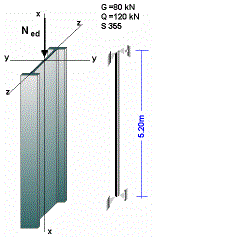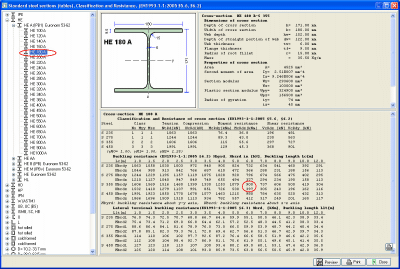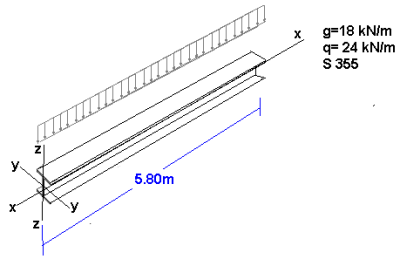# SteelSectionsEC3 Examples

## Examples

### Example 1Steel column 5.20 m.
Axial load G = 80 kN, variable axial load Q = 120 kN.
Steel S 355.

Ned =1.35xG+1.50xQ=1.35x80+1.50x120 = 288 kN
Buckling lengths: Liy = 5.20 m, Liz = 5.20 mIn the main program screen, click Classification andResistance of standard cross-sections.From the tree control on the left select section type HEA.
Click + and all the sections of type HEA are displayed.

For steel grade S 355 and buckling length 5.20 m (table values between 5.0 m and  6.0m ), check Nbyrd and Nbzrd (buckling resistances in compression in y-y and z-z axis) to be greater than the design load of the column Ned=288 kN.

Section
ΗΕ 180 Α is OK.

For buckling length 6.0m>5.20m, the section has, buckling resistances in compression Nbyrd = 900 kN > 288 kN and Nbzrd = 397 kN >288 kN.

### Example 2Variable load q = 24 kN/m.
Steel S 355.
qed = 1.35x18.0+1.50x24.0 = 60.30 kN/m
Maximum design bending moment:
My,ed =  60.30x5.802/8 = 253.6 kNm
Maximum design shearing force:

Vz,ed = 60.30x5.80/2 = 174.9 kN

In the main program screen, click Classification andResistance of standard cross-sections.From the tree control on the left select section type IPE. Click + and all the sections of type IPE are displayed.

For steel grade S 355 and lateral buckling length LLt=5.80 m (table 6.0m), check
Μbrd2 (parabolic bending moment diagram) to be greater than the maximum bending moment acting on the beam My,ed = 253.6 kNm.

Section IPE 500 is OK.

For lateral buckling length 6.0m>5.80 m, has resistance in bending moment due to lateral buckling
Μbrd2 = 288kNm>253.6 kNm
From the table above you can check the resistances in shear and bending.
Shear resistance Vc,rdz = 1227 kN, bending resistanceMc,rdy = 779 kNm.